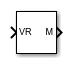# VR to MATLAB Coordinates

Convert VR coordinates to MATLAB coordinates

Since R2019a

•Libraries:

## Description

The VR to MATLAB Coordinates block converts a point with coordinates in the Virtual World coordinate system (Znear) to the MATLAB® coordinate system (Zup).

The following relation holds between the two coordinate systems:

[xm, ym, zm] = [xv, -zv, yv]

where MATLAB coordinates are denoted with the m subscript and Virtual World coordinates are denoted with the v subscript. For more information on the two coordinate systems, see Virtual World Coordinate System.

## Ports

### Input

expand all

Coordinates of a point in VRML notation, specified as a 3-element row vector.

Data Types: `single` | `double`

### Output

expand all

Coordinates of a point in MATLAB notation, returned as a 3-element row vector.

Data Types: `single` | `double`

## Version History

Introduced in R2019a